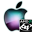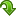ATOMS : Apifun details

# Apifun

Check input arguments in macros
Details
Version
0.4.3
Author
Michael Baudin
Owner Organization
- - -
Maintainers
Samuel Gougeon
Michael Baudin
Category
Creation Date
June 9, 2018
Source created on
Scilab 6.0.x
Binaries available on
Scilab 5.5.x:Windows 64-bitWindows 32-bitLinux 64-bitLinux 32-bitMacOSX
Scilab 6.0.x:Windows 64-bitWindows 32-bitLinux 64-bitLinux 32-bitMacOSX
Scilab 6.1.x:Windows 64-bitWindows 32-bitLinux 64-bitLinux 32-bitMacOSX
Install command
`--> atomsInstall("apifun")`
Report a bugDescription
```
This module aims at simplifying error checking when writing macros.

It provides a set of functions to check input arguments. Call to these functions
can replace duplicated, error-prone, boring "boiler-plate" code at the
beginning of the macros. They also make the error messages easy to localize.

For example, in functions with a variable number of input arguments, we often
have the following source code, which checks that  the number of input arguments
provided by the user is consistent with the number of expected arguments :

[lhs,rhs]=argn()
if ( rhs < 2 | rhs > 5 ) then
errmsg = msprintf(gettext("%s: Unexpected number of input arguments : %d
provided while from %d to %d are expected."), "myfunction",
rhs,2,5);
error(errmsg)
end

In this case, instead of the expected error, message tells the user that the
argument of the msprintf function has a wrong format ; it can confuse the user
about the source of the error.

Instead, only one call to the apifun_checkrhs function will correctly that the
number of input arguments provided by the user of the function corresponds to
the number of expected arguments. The following function takes 2/3 input
arguments and 1 output arguments:

function y = myfunction ( varargin )
[lhs, rhs] = argn()
apifun_checkrhs ( "myfunction" , rhs , 2:3 )
apifun_checklhs ( "myfunction" , lhs , 1 )
x1 = varargin(1)
x2 = varargin(2)
if ( rhs >= 3 ) then
x3 = varargin(3)
else
x3 = 2
end
y = x1 + x2 + x3
endfunction

When called with 1 or 4 arguments, an error will be generated.

// Calling sequences which work
y = myfunction ( 1 , 2 )
y = myfunction ( 1 , 2 , 3 )
// Calling sequences which generate an error
y = myfunction ( 1 )
y = myfunction ( 1 , 2 , 3 , 4 )

The following session shows the kind of error message which is produced by the
apifun module, for example when the number of input argument is wrong.
The error message is clear about the function which generates the message (e.g.
"myfunction").

-->y = myfunction ( 1 )
!--error 10000
myfunction: Unexpected number of input arguments : 1 provided while the number
of expected input arguments should be in the set [2 3].
at line     131 of function apifun_checkrhs called by :
at line       3 of function myfunction called by :
y = myfunction ( 1 )

The advantages of using the current module are the following.
* Designing robust functions is much simpler which improves both the
quality and the robustness of functions.
* The provided source code is factored into one single    module.
* It is similar to what is provided for gateways written in C/C++.

The following is a list of the function currently implemented in this module:

* apifun_overview — An overview of the Apifun toolbox.
* apifun_checklhs — Generates an error if the number of LHS is not in given
set.
* apifun_checkrhs — Generates an error if the number of RHS is not in given
set.

Support

* apifun_argindefault — Returns the value of an input argument.
* apifun_expandfromsize — Expand variables from a size.
* apifun_expandvar — Expand variables so that they all have the same shape.
* apifun_keyvaluepairs — Returns options from key-value pairs.

Check content

* apifun_checkcomplex — Generates an error if the variable has a zero
imaginary part.
* apifun_checkflint — Generates an error if the variable is not a floating
point integer.
* apifun_checkgreq — Check that the value is greater or equal than a
threshold.
* apifun_checkloweq — Checks that the value is lower or equal than a
threshold.
* apifun_checkoption — Generates an error if the value of an input argument
is not expected.
* apifun_checkrange — Check that the value is in a given range.
* apifun_checkreal — Generates an error if the variable has an imaginary
part.

Check size

* apifun_checkdims — Generates an error if the variable has not the required
size.
* apifun_checkscalar — Generates an error if the variable is not a scalar.
* apifun_checksquare — Generates an error if the variable is not a square
matrix.
* apifun_checkveccol — Generates an error if the variable is not a column
vector.
* apifun_checkvecrow — Generates an error if the variable is not a row
vector.
* apifun_checkvector — Generates an error if the variable is not a vector.

Check type

* apifun_checkcallable — Generates an error if the variable is not a
callable
function.
* apifun_checktype — Generates an error if the given variable is not of
expected type.
```Files (4)OS-independent binary for Scilab 5.5.x
```Binary for Scilab 5.5
```OS-independent binary for Scilab 6.0.x
```Binary for Scilab 6.0
```OS-independent binary for Scilab 6.1.x
```
```Source code archive
```
```News (0)Comments (0)Leave a comment
You must register and log in before leaving a comment.Email notifications
Send me email when this toolbox has changes, new files or a new release.